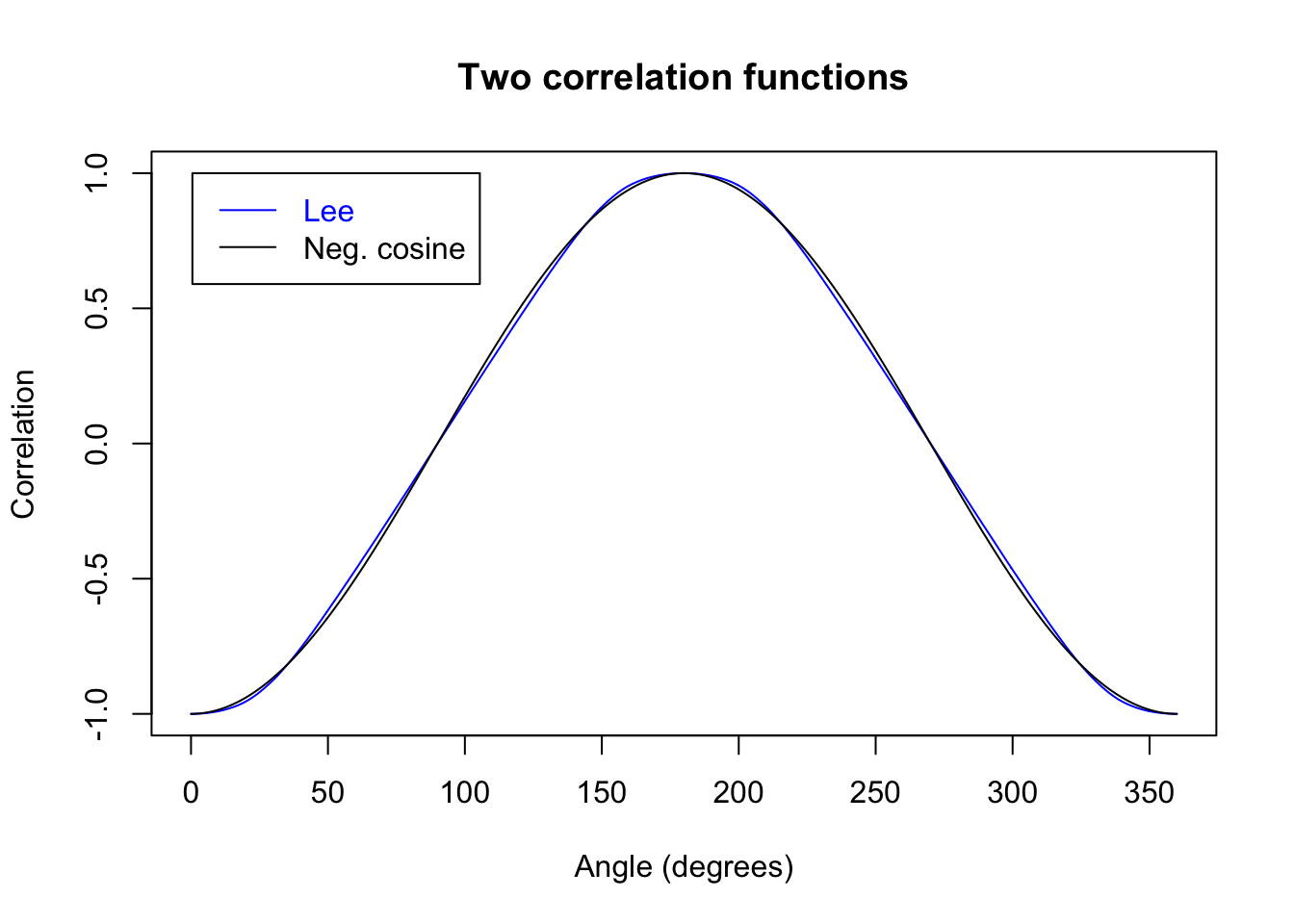## A simulation of Justin Lee's (2014) precession model for the EPR-Bohm correlations
## http://vixra.org/abs/1408.0063 "Bell's Inequality Loophole: Precession"

## There is some discussion of this paper together with an earlier paper on PubPeer
## https://pubpeer.com/publications/2C868EDB0FB35A23005FF34F50ED90
## "EPR Paradox Solved by Special Theory of Relativity"
## J. Lee, Acta Physica Polonica A (2014)
## http://vixra.org/abs/1306.0184

## For reproducibility. Replace integer seed by your own, or delete this line
## and let your computer dream up one for you (system time + process ID).

set.seed(9875)

## Uniform random points on sphere generated by Dave Seaman's trig method':
## http://www.math.niu.edu/~rusin/known-math/96/sph.rand (method 3)
## http://mathforum.org/kb/message.jspa?messageID=393612
## http://rpubs.com/gill1109/13340 has an R illustration

## Choose z uniformly distributed in [-1,1]
## Choose t uniformly distributed on [0, 2*pi)
## Let r = sqrt(1-z^2)
## Let x = r * cos(t)
## Let y = r * sin

## The function "sphereSample" returns a 3 x M matrix.
## Its M columns represent the x, y and z coordinates
## of M uniform random points on the sphere S^2

sphereSample <- function(){
z <- runif(M, -1, 1)
t <- runif(M, 0, 2*pi)
r <- sqrt(1 - z^2)
x <- r * cos(t)
y <- r * sin(t)
rbind(x, y, z)
}

M <- 10^6  ## Sample size

## Measurement angles for setting 'a': directions in the equatorial plane
angles <- seq(from = 0, to = 360, by = 0.5) * 2 * pi/360

K <- length(angles)
corrs <- numeric(K)  ## Container for correlations
Ns <- numeric(K)  ## Container for number of states

beta <- 0 * 2 * pi/360  ## Measurement direction 'b' fixed, in equatorial plane

e <- sphereSample()  # the common spin direction shared by each particle
f <- sphereSample()  # this will generate the random precession of particle 1
g <- sphereSample()  # this will generate the random precession of particle 2

theta <- 10   # this is the angular separation between the precessing spins
# and their central values e and - e (degrees).
# 10 degrees, as in Lee, Figure 1d
C <- cos(theta * 2 * pi / 360)
S <- sin(theta * 2 * pi / 360)

delta <- 10    # this is the detection threshhold, as an angular separation
# between the precessing spins and the plane orthogonal
# to the measurement direction (degrees)
# 10 degrees, as in Lee, Figure 1d
D <- sin(delta* 2 * pi / 360)

vec2mat <- function(x) matrix(x, 3, M, byrow = TRUE)

fPerp <- f - vec2mat(colSums(e * f)) * e
# Projecting particle 1's precession vector f, to be orthogonal to e
fPerpNorm <- fPerp / vec2mat(sqrt(colSums(fPerp*fPerp)))
# Normalising to length 1

gPerp <- g - vec2mat(colSums(e * g)) * e
# Projecting particle 2's precession to be orthogonal to e
gPerpNorm <- gPerp / vec2mat(sqrt(colSums(gPerp*gPerp)))
# Normalising to length 1

AliceSpins <- C * e + S * fPerpNorm # add precession vector to "true" spin
BobSpins <- - C * e + S * gPerpNorm # add independent precession vector to "true" spin

b <- c(cos(beta), sin(beta), 0)  ## Measurement vector 'b'
cb <- colSums(BobSpins * b)  ## Inner products of cols of 'BobSpins' with 'b'
NSingles <- sum(abs(cb) > D)

## Loop through measurement vectors 'a' (except last = 360 degrees = first)

for (i in 1:(K - 1)) {
alpha <- angles[i]
a <- c(cos(alpha), sin(alpha), 0)  ## Measurement vector 'a'
ca <- colSums(AliceSpins * a)  ## Inner products of cols of 'AliceSpins' with 'a'
good <- abs(ca) > D & abs(cb) > D
## Select particle pairs whose spins are not too nearly orthogonal to measurement directions
N <- sum(good)
corrs[i] <- sum(sign(ca[good]) * sign(cb[good]))/N
Ns[i] <- N
}

corrs[K] <- corrs
Ns[K] <- Ns

plot(angles * 180/pi, corrs, col = "blue", main = "Two correlation functions",
xlab = "Angle (degrees)", ylab = "Correlation", type = "l")

lines(angles * 180/pi, - cos(angles), col = "black")

legend(x = 0.5, y = 1.0, legend = c("Lee", "Neg. cosine"),
text.col = c("blue", "black"), lty = 1, col = c("blue", "black"))## Critical correlations

CHSHangles <- c(45, 135, 225, 315)
CHSHindices <- integer(4)
for (i in 1:4) CHSHindices[i] <- (1:K) [abs(angles * 180 / pi - CHSHangles[i]) < 0.1]

table1 <- corrs[CHSHindices]
names(table1) <- CHSHangles
table1
##         45        135        225        315
## -0.6892271  0.6888248  0.6892271 -0.6888248
# Compare with singlet

table1bis <- - cos(CHSHangles * pi / 180)
names(table1bis) <- CHSHangles
table1bis           
##         45        135        225        315
## -0.7071068  0.7071068  0.7071068 -0.7071068
plot(angles * 180/pi, Ns/NSingles, type = "l", main = "Apparent efficiency",
xlab = "Angle (degrees)", ylab = "Ratio coincidences to singles")## Critical efficiencies (coincidences to singles)
table2 <- (Ns/NSingles)[CHSHindices]
names(table2) <- CHSHangles
table2
##        45       135       225       315
## 0.8251215 0.8242490 0.8251215 0.8242490
plot(angles * 180/pi, Ns/M, type = "l", main = "Global pair acceptance rate",
xlab = "Angle (degrees)", ylab = "Ratio accepted pairs to all pairs")## Critical efficiencies (pair acceptance rate)

table3 <- (Ns/M)[CHSHindices]
names(table3) <- CHSHangles
table3
##       45      135      225      315
## 0.681887 0.681166 0.681887 0.681166